Maths-
General
Easy

Question

# If there is an error of 0.02 sq. cm is made in calculating the area of a circle. If the radius is 5cm, then the percentage error in the circumference of the circle is

##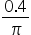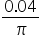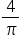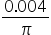Hint:

## The correct answer is:### Given :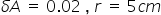Area of circle(A) =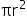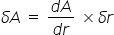Differentiating 'A' w.r.t 'r'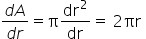0.02 =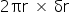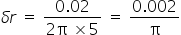Circumference of circle(C) =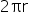C = 2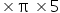C = 10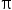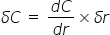Differentiating 'C' w.r.t 'r'% error in the circumference of the circle =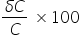% error in the circumference of the circle  =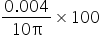=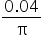null>#### With Turito Foundation.#### Get an Expert Advice From Turito.